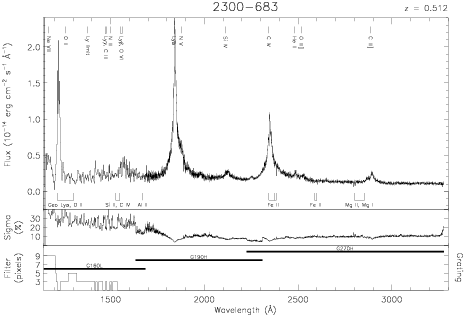Download dataset
TELESCOP= 'HST     '
INSTRUME= 'FOS     '
RADECSYS= 'FK5     '
EQUINOX =               2000.0
DATE-OBS= '1992-06-16T10:54:42'
RA_PNT  =       345.9312500000
DEC_PNT =       -68.1269722222
PA_PNT  =        13.0120209861
OBJECT  = '2300-683'
ROOTNM01= 'Y0RV1O03T'
GRNDMD01= 'RAPID-READOUT'
DETECT01= 'AMBER   '
APERID01= 'C-2     '
FGWAID01= 'H19     '
ROOTNM02= 'Y0RV1O04T'
GRNDMD02= 'RAPID-READOUT'
DETECT02= 'AMBER   '
APERID02= 'C-2     '
FGWAID02= 'H19     '
ROOTNM03= 'Y0RV1O05T'
GRNDMD03= 'RAPID-READOUT'
DETECT03= 'AMBER   '
APERID03= 'C-2     '
FGWAID03= 'H27     '
ROOTNM04= 'Y0RV1O06T'
GRNDMD04= 'RAPID-READOUT'
DETECT04= 'BLUE    '
APERID04= 'B-3     '
FGWAID04= 'L15     '